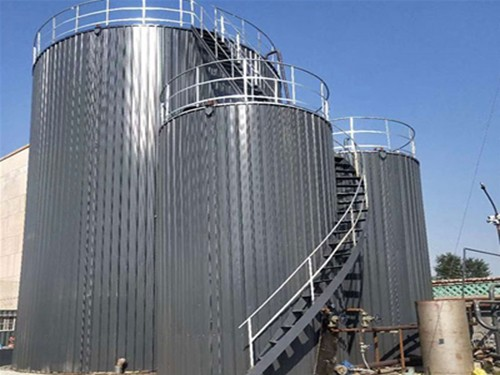13869248267

# 沥青罐与沥青高温罐的选用方法

m=P·h·k=80×10×5%=40

M=A·m=3×40=120

Q大=Q储保+Q高保+Q高加式中Q大----加热，保温所需热量，kj/h，Q储保----储罐沥青保温所需热量，kj/h，Q高保----高温罐沥青保温所需热量，kj/h，Q高加----高温罐沥青加温所需热量，kj/h。

Q储保=M储·C储·Δt=50×10³×1.6×2=1.6×105kj/h

Q高保=M高·C高·Δt=10×10³×2.1×2=0.42×105kj/h

Q高加==M·C（t1-t2）/b+KF（ty-t0）/s=10×10³×1.7(170-100)/2+10×30(135-10)/2=6.14×105kj/h

Q大=8.16×105kj/h

Q´=Q大/B=8.16×105/0.75=26×104kCal/h#### 【相关推荐】

• 联系电话

13869248267
• 微信扫一扫